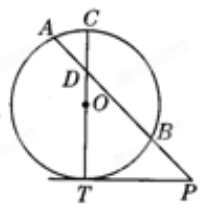PythagoreanTheorem PowerOfPointTheorem

Problem - 3143

Line $PT$ is tangent to circle $O$ at point $T$. $PA$ intersects circle $O$ and its diameter $CT$ at $B$, $D$, and $A$ in that order. If $CD=2, AD=3,$ and $BD=6$, find the length of $PB$.